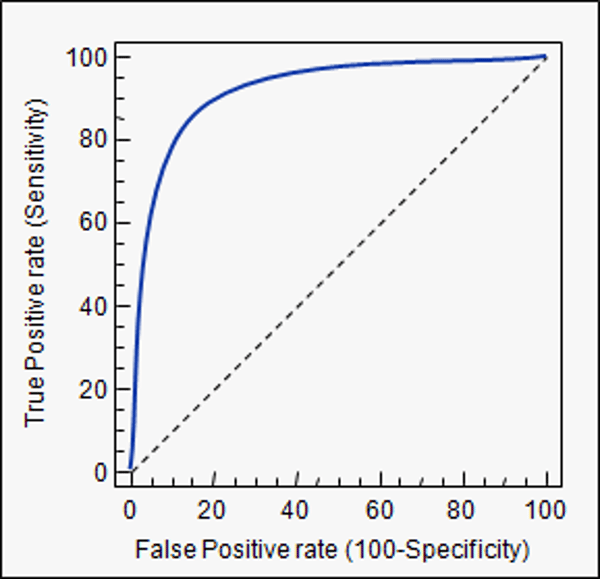Trusted answers to developer questions
Trusted Answers to Developer Questions

Related Tags

roc
curve
machine
learning

# What are ROC curves?Educative Answers Team

Grokking Modern System Design Interview for Engineers & Managers

Ace your System Design Interview and take your career to the next level. Learn to handle the design of applications like Netflix, Quora, Facebook, Uber, and many more in a 45-min interview. Learn the RESHADED framework for architecting web-scale applications by determining requirements, constraints, and assumptions before diving into a step-by-step design process.

Receiver Operating Characteristic (ROC) curves summarize the trade-off between the true positive rate (TPR) and the false positive rate (FPR) for a predictive model using different probability thresholds.

ROC curves are appropriate when observations are balanced between each class in the dataset.Sample ROC curve.

The ROC curve is plotted with TPR (also represented as Sensitivity) against the FPR (also represented as 1 $-$ Specificity) where TPR is on the y-axis and FPR is on the x-axis. The formula to calculate these values is:

$TPR = Sensitivity = True Positives / (True Positives + False Negatives)$ and $FPR = 1 - Specificity = True Negatives / (True Negatives + False Positives)$

The ROC curve is a useful tool because:

• The curves of different models can be compared directly in general.
• The area under the curve (AUC) can be used as a summary of the model skill.

RELATED TAGS

roc
curve
machine
learning